DEFINITIONS
The "Physics of Petrophysics" is the dominant theme of this Chapter, which covers most of the underlying principles. Here we provide the basic physical concepts required to predict log analysis parameters for gases, liquids, solids, and mixtures. Much of this material was covered in high-school and university level courses in Physics, but the application to rocks containing economic minerals might have been overlooked. A few initial definitions are in order.

A law can be proved, using the most primitive of physical or mathematical rules, whereas a theory cannot be proved. For example, the Law of Conservation of Energy can be proved by invoking more primitive physical laws. The Theory of Relativity cannot yet be proved, and alternate theories exist, although they are not widely held.

A good theory explains all the known data, and may even predict as yet unobserved data, as the Theory of Relativity has done. A poor theory may still be widely believed, even if it fails to account for all observed facts. Some believers may discount the data that does not fit, assuming it is in error, or will predict that improvements to the theory will allow all data to fit. The controversy over Creation (now known as Intelligent Design) versus Evolution falls into this category.

An empirical relationship differs from both a law and a theory. The empirical relationship is a mathematical "best fit" between two or more observed sets of data. Many individual data sets will not follow the empirical relationship well. For example, it is often true that a larger object weighs more than a smaller item, but there are many exceptions to that rule.

These relationships are often termed rules of thumb, and frequently apply only in limited areas or under very restrictive circumstances. Some relationships used in log analysis are actually laws, such as those dealing with the summation of densities in mixtures. Many, if not most, are empirical relationships, such as the Wyllie time-average formula, or the Archie formation factor concept.PARTICLE PHYSICS
For more than a century, we were taught that the elementary particles of matter were positively charged protons, neutral neutrons, and negatively charged electrons. All matter in the universe was thought to be made up of stable, and some unstable, combinations of these three particles, forming larger particles called atoms. The particles are held together to form elements by forces of attraction between the basic particles.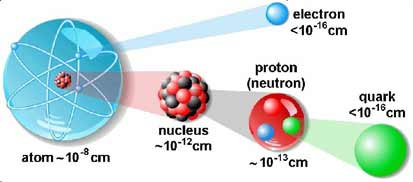Basic particles are pretty small. For comparison, the Universe is 10^28, the
Milky Way is 10^23, the Solar System is 10^15, and Earth is 10^9 cm in diameter

More recently, nuclear physicists have proposed the "Standard Model", showing that these so-called "basic particles" are actually made of even smaller elementary particles called, naturally enough, sub-atomic particles. There are two types of subatomic particles: elementary particles, which are not made of other particles, and composite particles.

Some scientists have postulated that these elementary particles are composed of even more basic particles called preons (not to be confused with prions, a type of protein). No evidence exists to support this conjecture.

The elementary particles of the Standard Model include:
* Six "flavours" of Quarks: up, down, bottom, top, strange, and charm
* Six types of Leptons: electron, electron neutrino, muon, muon neutrino, tau, tau neutrino
* Thirteen gauge Bosons (force carriers): the graviton of gravity, the photon of electromagnetism,
the three W+, W--  and Z bosons of the weak force, and the eight gluons of the strong force.

Composite particles include all hadrons, subdivided into two categories:  Baryons and Mesons. Composite subatomic particles (such as protons or atomic nuclei) are bound states of two or more elementary particles. For example, a proton is made of two up quarks and one down quark, while the atomic nucleus of helium-4 is composed of two protons and two neutrons.Hadrons are any strongly interacting composite subatomic particle. All hadrons are composed of quarks.Baryons are strongly interacting fermions such as neutrons and protons, made up of three quarks.Mesons are strongly interacting bosons consisting of a quark and an antiquark.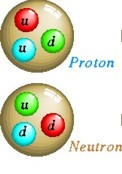QUARKS    Abbrev   Elec Charge    Mass
Up                    u           +2/3               2 MeV    Stable
Down               d            -1/3               5 MeV    Stable
Two Up quarks and 1 Down quark make a Proton with net charge of +1.
Two Down quarks and 1 Up quark make a Neutron with net charge of  0.

Charm             C             +2/3            1.25 GeV   Unstable
Strange           S             - 1/3              95  MeV   Unstable
Top                  t             +2/3              171 GeV   Unstable
Bottom            b              -1/3              4.2 GeV   Unstable
The unstable quarks make up short-lived particles, seen only in very high energy physics labs and cosmic rays.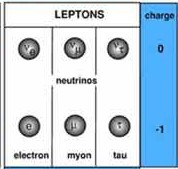LEPTONS   Abbrev   Elec Charge    Mass
Electron                e             -1                0.511 MeV    Stable
Muon                    u             -1                 105 MeV      Unstable
Tau                       T             -1                 1.78 GeV     Unstable e .

There are three Neutrinos corresponding to each of the three leptons. Neutrinos have no charge and rarely interact with ordinary matter.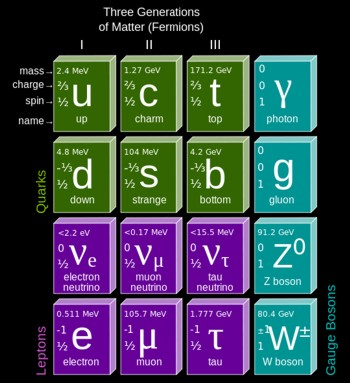Antiparticles equivalents to the quarks and leptons exist, such as positrons, antiprotons, or antineutrons, having the same mass, average lifetime, spin, magnitude of magnetic moment, and magnitude of electric charge as the particle to which they correspond, but having the opposite sign of electric charge, opposite intrinsic parity, and opposite direction of magnetic moment. They exist today only in high energy particle accelerators but were abundant, in theory, in the early moments of the Big Bang .Fermions comprise all particles with spin of 1/2. These are the 6 quarks, 6 anti-quarks, 6 leptons, and 6 anti-leptons.

Period Table of Quarks, Leptons, and Bosons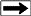Photons carry electoomagnetic energy, such as light, radio waves, and gamma rays.Gluons come in eight different species. They carry the strong force that binds quarks into other particles.Bosons carry the forces that act to bind or attract particles. The most obvious boson is the photon, the carrier of electromagnetic radiation (eg: light, radio, television, gamma rays, X-rays). Photons can have an effect over huge distances. Photons can behave as particles or waves, leading to a duality that underlies much of quantum physics.

The Z boson, W- boson, and W+ boson operate over very tiny inter-atomic distances (10^-18 meters), carrying the weak force.

The Higgs boson (graviton), postulated to carry the force of gravity, may have been discovered in 2012 at the Large Hadron Collider at CERN in Geneva. If it exists, theory predicts that it has a mass greater than 125 Gev.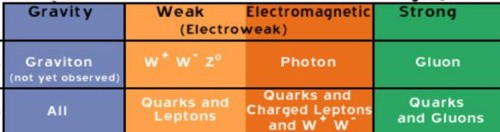Particles carry the weak and strong forces

 DARK ENERGY Dark Energy is a hypothetical form of energy that permeates all of space and tends to increase the rate of expansion of the universe. It is the most popular way to explain observations that the universe appears to be expanding at an accelerating rate. In the standard model of cosmology, dark energy currently accounts for 74% of the total mass-energy of the universe.Dark Matter involves heavy but virtually undetectable particles called neutralinos (not to be confused with neutrinos). Neutralinos need a mass of 100 to 1000 times that of a proton. They are also called weakly interacting massive particles (WIMPs) and have not been detected directly.

Another model proposes a different particle, the axion, that is one trillionth the mass of an electron. It will take quite a few of them to make up the missing mass.

Dark matter is weakly or non-interacting, so it is called nonbaryonic matter. It's composition is as yet unknown.Atoms consists of at least one proton and one electron (hydrogen). The nucleus of all other atoms consists of protons and neutrons, surrounded by electrons.Elements are made of one or more atoms with the same number of protons. An element cannot be broken into smaller elements by ordinary chemical processes. Helium, oxygen, sodium or chlorine are elements. There are 117 elements known to date, the heaviest being unstable and very short-lived. Unstable elements are said to be radioactive, decaying in time to some lighter, more stable element.Atomic Number (Z) represents the number of protons in an atom and uniquely identifies a chemical element. The number of electrons surrounding the nucleus equals the number of protons.Atomic Weight (A), or Mass Number, is the number of protons plus neutrons in the nucleus of an atom.Isotopes of an element have the same number of protons and electrons (same Atomic Number Z), but different numbers of neutrons. Some isotopes are stable, some are radioactive. About 339 isotopes occur naturally on Earth, of which about 79% are stable. Counting the radioactive isotopes not found in nature that have been created artificially, more than 3100 are currently known.

Unstable isotopes decay to more stable forms, some of which may be unstable and decay further. The decay process gives off radiation. The time it takes for the unstable material to decay to one half its original mass is called the half life.

For example, 93% of potassium atoms have 19 protons with 20 neutron and are stable, giving an atomic number of 19 and an atomic weight of 39. One particular isotope has 21 neutrons, giving an atomic weight of 40. It is unstable and comprises only 0.012% of all Potassium atoms. Other isotopes, some stable, some not, make up the remaining 7% of the atoms.RADIOACTIVITY
The common forms of radioactive decay are by emitting Alpha,  Beta, or Gamma particles (or rays), There are other more complicated forms of radioactivity not described here, for example various methods of inducing neutron emissions for use in medicine or well logging.

Atoms in a radioactive substance decay in a random fashion but at a characteristic rate. The length of time this takes, the number of steps required, and the kinds of radiation released at each step are well known from laboratory measurements and quantum theory calculations.Half-Life is the time taken for half of the atoms of a radioactive substance to decay. Half-lives can range from less than a millionth of a second to millions of years depending on the element concerned. After one half-life the level of radioactivity of a substance is halved, after two half-lives it is reduced to one quarter, after three half-lives to one-eighth and so on.Alpha Decay is a type of radioactive decay in which two protons and two neutrons are emitted. They are bound together into a particle identical to a helium nucleus. The original atom transforms into an atom with a mass number 4 less and atomic number 2 less than the original atom. A common example is the decay of Uranium-238 into Thorium-234. Two electrons are also stripped from the original atom.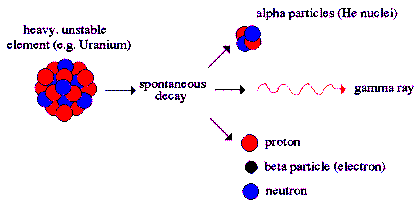Alpha radiation produces a helium atom and one or more gamma rays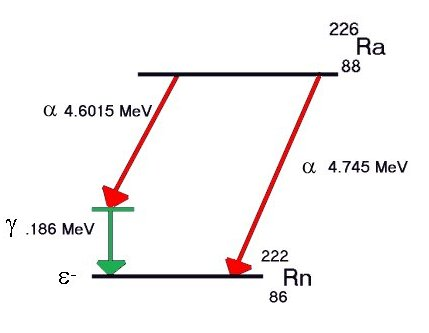There is a difference in mass between the original nucleus and the sum of the mass of the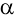particle and resulting nucleus. This lost mass is converted into energy using the formula E = mc2; the energy would equal the kinetic energy of theparticle and the recoil energy of the resulting nucleus. Excess energy, if any, is released as one or more gamma rays. For example, some Radium decays to Radon give gamma rays, some do not. Some alpha events never produce gamma rays.

Alpha particles cannot travel far and are not particularly dangerous unless ingested.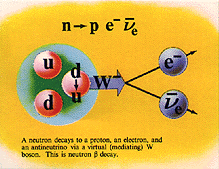Beta Emission is a process in which an electron and an antineutrino (or a positron and a neutrino) are emitted. In the case of electron emission, a neutron is converted to a proton and is referred to as "beta-minus", and the atomic number is increased by 1. No gamma rays are emitted .

In the case of a positron emission, a proton is converted into a neutron and is called "beta plus". The positron is quickly annialated by a nearby electron and two gamma rays are emitted. the atomic number is decreased by 1.

For Potasium-40, beta-minus results in Calcium-40 and Beta-plus results in Argon-40. Both daughter products are stable. Gamma rays are produced in Beta-plus but not Beta-minus events.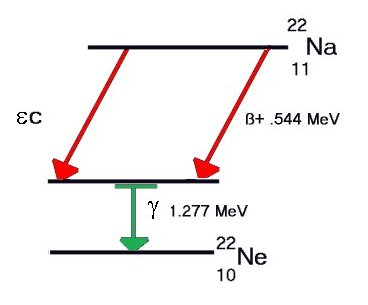A third form of Beta decay, called Inverse Beta, or Electron Capture, converts a proton to a neutron by capturing an inner shell electron, and emitting the excess energy as a low energy gamma ray (X-ray). For Potassium-40, this mode of decay also results in stable Argon-40. Since K-40 has a half-life of more than a billion years, gamma rays are constantly being produced and can be detected by conventional instrumentation.

Some elements decay to a stable form through a series of alpha and beta emissions, the longest chain being that for uranium, shown below. Note that each Beta-minus decay increases the atomic number by 1 and each Alpha decay decreases it by 2.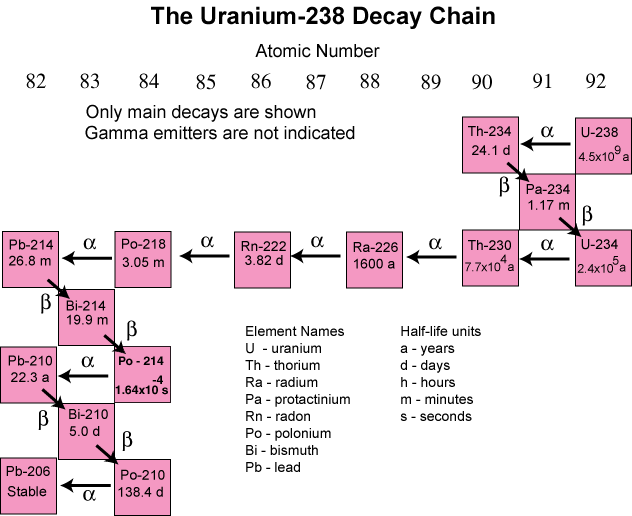Decay chain for Uranium-238 to stable Lead-206Gamma Rays are high energy photons, a form of electromagnetic radiation, produced by sub-atomic particle interactions, such as electron-positron annihilation or radioactive decay. Gamma rays are generally characterized as having the highest frequency and energy, and also the shortest wavelength (below about 10 picometers).

Hard X-rays overlap the range of long-wavelength (lower energy) gamma rays, however the distinction between the two terms depends on the source of the radiation, not its wavelength; X-ray photons are generated by energetic electron processes, gamma rays by transitions within atomic nuclei. Due to their high energy content, X-rays and gamma rays can cause serious damage when absorbed by living cells.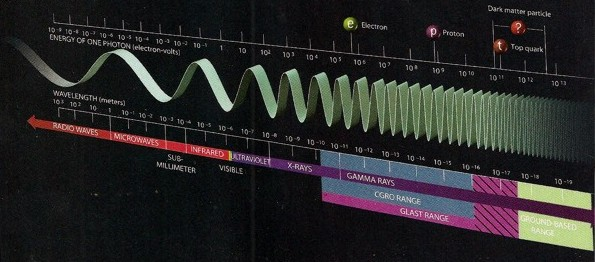Energy and frequency of major sub-atomic particles (click image for larger view)

In well logging, the natural gamma radiation from rocks is used to to assist in assessing mineralogy of the rocks. Another well logging tool emits neutrons, either from a chemical or accelerator source in the logging tool, to help assess mineralogy and porosity. Instruments that emit gamma rays are also used for similar purposes.Photo-electric Effect occurs when a low energy photon, such as light, strikes an electron in an atom. The photon is absorbed and an electron is ejected from the atom, provided the photon has sufficient energy. The phenomenon was described by Hertz and others in the late 1800's and by Einstein in 1905. Einstein's experiments demonstrated the quantum nature of photons.Compton scattering was described in 1923. High energy photons, such as gamma rays, react somewhat differently rhan low energy photons. In this case, the photon kicks out one electron, but the photon continues moving on at a lower energy. The fact that the photon loses energy demonstrates its wave-like characteristics.

The photoelectric effect takes place with photons with energies from about a few electronvolts to over 1 MeV. At higher photon energies comparable to the electron rest energy of 511 keV, Compton scattering takes place, and above twice this (1.022 MeV) pair production takes place. However, like all radioactive events, these reactions are statistical in nature, so there is no distinct energy boundary between the three processes.

In well logging, Compton scattering of gamma rays is used to measure the electron density of rocks. This is transformed into density in grams/cc. At the same time, the energy of the scattered gamma rays is measured and transformed into a value called the Photo Electric Effect (PEF), This is a little confusing since the measurement is made from scattered gamma rays, and not from a direct measure of the ejected electrons, as was done in the 1905 Einstein experiment.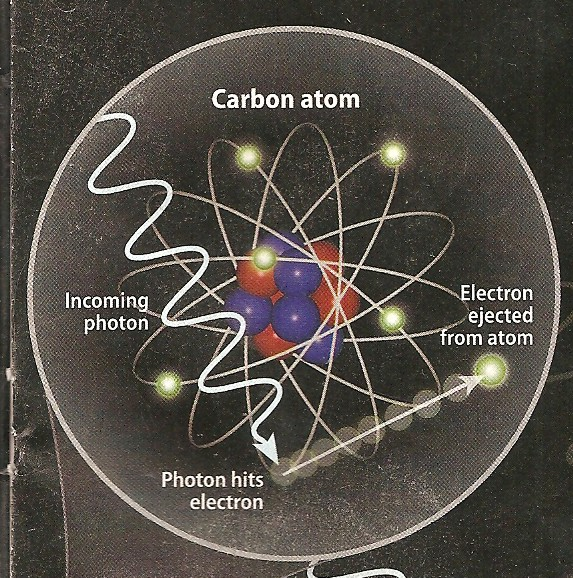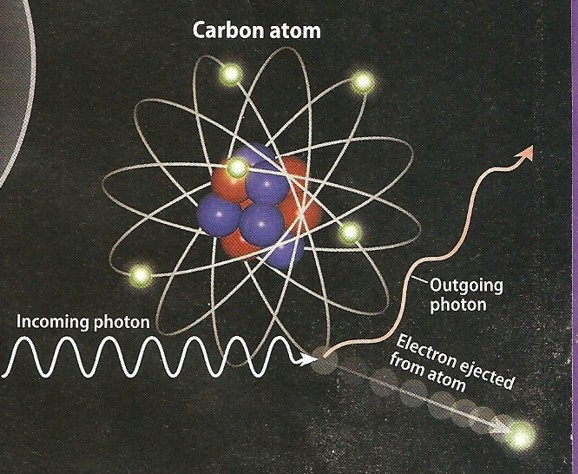Photo electric effect (left)  and Compton scattering (right) show low energy and higher energy reactions to impinging photons.ANIMATIONS
FROM: ThinkQuest.com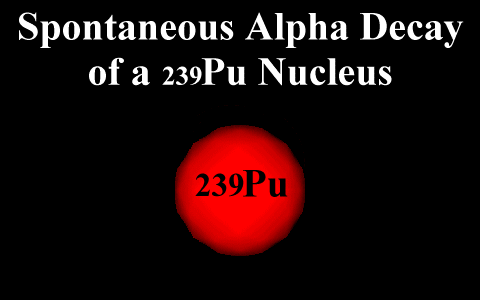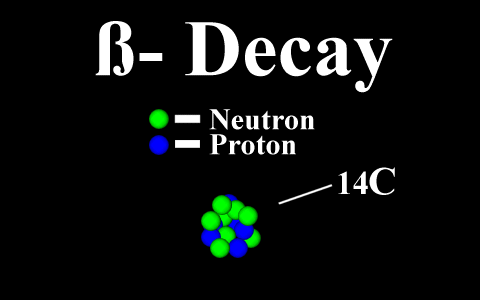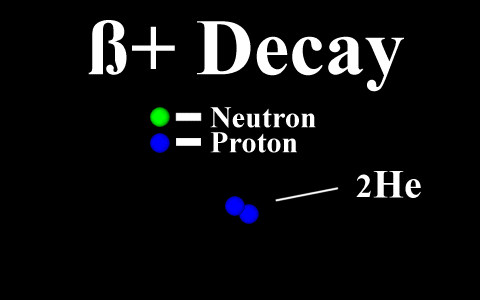Page Views ---- Since 01 Jan 2015
Copyright 2023 by Accessible Petrophysics Ltd.
CPH Logo, "CPH", "CPH Gold Member", "CPH Platinum Member", "Crain's Rules", "Meta/Log", "Computer-Ready-Math", "Petro/Fusion Scripts" are Trademarks of the Author